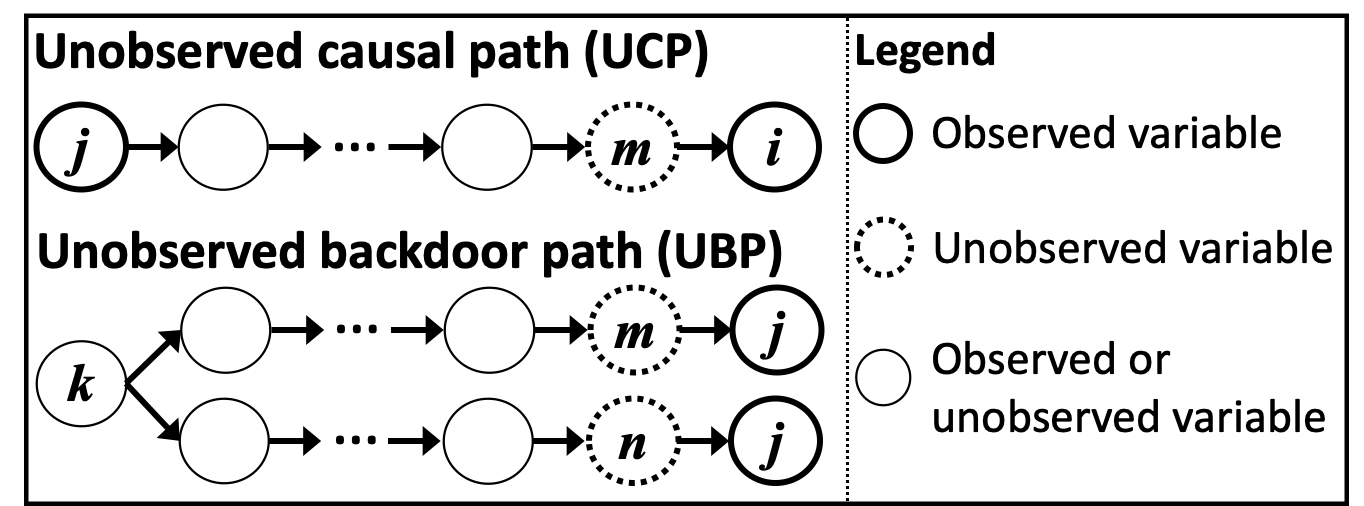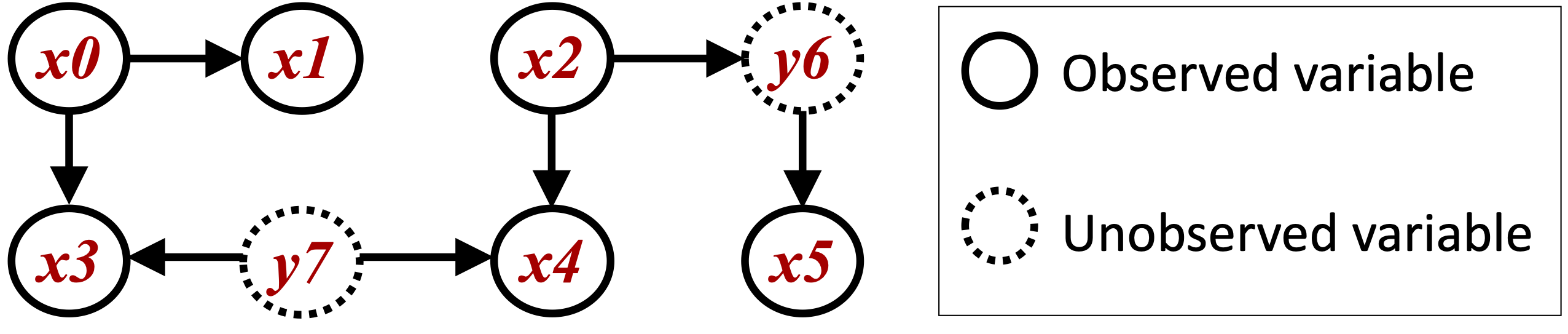# CAM-UV

## Model

This method CAM-UV (Causal Additive Models with Unobserved Variables) assumes an extension of the basic CAM model  to include unobserved variables. This method makes the following assumptions:

1. The effects of direct causes on a variable form generalized additive models (GAMs).

2. The causal structures form directed acyclic graphs (DAGs).

CAM-UV allows the existence of unobserved variables. It outputs a causal graph where a undirected edge indicates the pair of variables that have an unobserved causal path (UCP) or an unobserved backdoor path (UBP), and a directed edge indicates the causal direction of a pair of variables that do not have an UCP or UBP.

Definition of UCPs ans UBPs: As shown in the below figure, a causal path from $$x_j$$ to $$x_i$$ is called an UCP if it ends with the directed edge connecting $$x_i$$ and its unobserved direct cause. A backdoor path between $$x_i$$ and $$x_j$$ is called an UBP if it starts with the edge connecting $$x_i$$ and its unobserved direct cause, and ends with the edge connecting $$x_j$$ and its unobserved direct cause.References

## Import and settings

In this example, we need to import numpy in addition to lingam.

import numpy as np
import random
import lingam


## Test data

First, we generate a causal structure with 2 unobserved variables (y6 and y7) and 6 observed variables (x0–x5) as shown in the below figure.def get_noise(n):
noise = ((np.random.rand(1, n)-0.5)*5).reshape(n)
mean = get_random_constant(0.0,2.0)
noise += mean
return noise

def causal_func(cause):
a = get_random_constant(-5.0,5.0)
b = get_random_constant(-1.0,1.0)
c = int(random.uniform(2,3))
return ((cause+a)**(c))+b

def get_random_constant(s,b):
constant = random.uniform(-1.0, 1.0)
if constant>0:
constant = random.uniform(s, b)
else:
constant = random.uniform(-b, -s)
return constant

def create_data(n):
causal_pairs = [[0,1],[0,3],[2,4]]
intermediate_pairs = [[2,5]]
confounder_pairs = [[3,4]]

n_variables = 6

data = np.zeros((n, n_variables)) # observed data
confounders = np.zeros((n, len(confounder_pairs))) # data of unobserced common causes

for i in range(n_variables):
data[:,i] = get_noise(n)
for i in range(len(confounder_pairs)):
confounders[:,i] = get_noise(n)
confounders[:,i] = confounders[:,i] / np.std(confounders[:,i])

# Adding the effects of unobserved common causes
for i, cpair in enumerate(confounder_pairs):
cpair = list(cpair)
cpair.sort()
data[:,cpair] += causal_func(confounders[:,i])
data[:,cpair] += causal_func(confounders[:,i])

for i1 in range(n_variables)[0:n_variables]:
data[:,i1] = data[:,i1] / np.std(data[:,i1])
for i2 in range(n_variables)[i1+1:n_variables+1]:
# Adding direct effects between observed variables
if [i1, i2] in causal_pairs:
data[:,i2] += causal_func(data[:,i1])
# Adding undirected effects between observed variables mediated through unobserved variables
if [i1, i2] in intermediate_pairs:
interm = causal_func(data[:,i1])+get_noise(n)
interm = interm / np.std(interm)
data[:,i2] += causal_func(interm)

return data

sample_size = 2000
X = create_data(sample_size)


## Causal Discovery

To run causal discovery, we create a CAMUV object and call the fit method.

model = lingam.CAMUV()
model.fit(X)


Using the adjacency_matrix_ properties, we can see the adjacency matrix as a result of the causal discovery. When the value of a variable pair is np.nan, the variables have a UCP or UBP.

model.adjacency_matrix_

array([[ 0.,  0.,  0.,  0.,  0.,  0.],
[ 1.,  0.,  0.,  0.,  0.,  0.],
[ 0.,  0.,  0.,  0.,  0., nan],
[ 1.,  0.,  0.,  0., nan,  0.],
[ 0.,  0.,  1., nan,  0.,  0.],
[ 0.,  0., nan,  0.,  0.,  0.]])